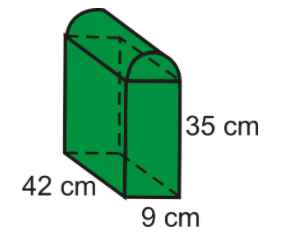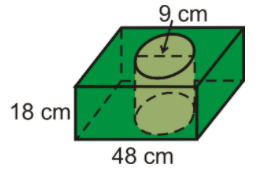# Problems on ratio, rate, proportion, scale drawings, maps

### Questions

For the following 3 figures, find the volume and total surface area.

1. In the following figure, in addition to the dimensions labelled in the diagram, the “slanted” height of the “roof” is 28.8 cm.2.3. The following figure is made by removing a cylinder of diameter 9 cm and height 18 cm from a cuboid with a square base of sides 48 cm and height 18 cm.2. The petrol consumption of Mr Lim’s car is 8 km per litre. Each week, he has to travel an average distance of 420 km. If petrol is charged at $1.40 per litre, what is Mr Lim’s average weekly petrol bill? 3. A runner took 2 hr 34 min to finish a 42-km marathon. Assuming that he ran at a constant speed, how long did he take to run 15 km? 4. A rope is cut into two pieces in the ratio$3:2$. The longer piece is 9.6 m long. Find the length of the shorter piece. 5. A sum of money is divided in the ratio 4:3. The larger part is$7.20. Find the smaller part.
6. Express the ratio 700 g to 1.75 kg in its lowest terms. Give your answer in the form $m:n$, where $m$ and $n$ are integers.
7. A map is drawn to a scale of $1 : 400 \; 000$.